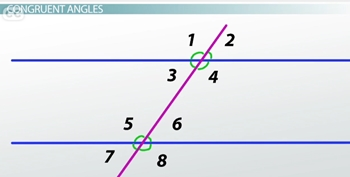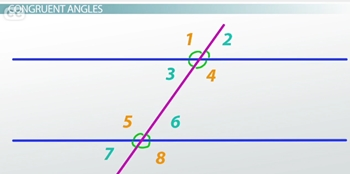# Angles Formed by a Transversal

An error occurred trying to load this video.

Try refreshing the page, or contact customer support.

Coming up next: Parallel Lines: How to Prove Lines Are Parallel

### You're on a roll. Keep up the good work!

Replay
Your next lesson will play in 10 seconds
• 0:05 Parallel Lines
• 0:37 The Transversal
• 1:03 The Angles
• 1:40 Congruent Angles
• 6:22 Lesson Summary

Want to watch this again later?

Timeline
Autoplay
Autoplay
Speed

#### Recommended Lessons and Courses for You

Lesson Transcript
Instructor: Yuanxin (Amy) Yang Alcocer

Amy has a master's degree in secondary education and has taught math at a public charter high school.

When you have a pair of parallel lines and a transversal, something very interesting happens to the angles that are formed. You can see this happen in real life at street intersections and such. Watch this video lesson to learn about all of this.

## Parallel Lines

Before we have a transversal, we need a pair of parallel lines. These types of lines will never intersect and are always the same distance apart. In some cities, where the blocks are rectangles or squares, the streets generally run parallel to each other. If you are one street, there is no way you can get to the street parallel to yours without using a street that runs in a different direction.

## The Transversal

This street that runs in a different direction can be likened to the transversal, a straight line that crosses two other lines. In this particular video lesson, we are going to talk about our transversal crossing a pair of parallel lines. I want to show you how, in this situation, something interesting happens to the angles that are formed by it.

## The Angles

When a transversal crosses a pair of parallel lines, you end up with a total of 8 angles. Before I tell you what that interesting thing is, let me label these angles so that we can talk about them better. I'm going to label each angle with a number starting with the number 1 on the upper left and then working my way down to angle 8 on the lower right.You might already be able to guess what that interesting thing is when you look carefully at the different angles.

## Congruent Angles

What happens is that when you have a transversal crossing a pair of parallel lines, you end up with only two different-sized angles. I know there are 8 angles in total, but you can match them up into two groups of four equal angles. There are also four different ways to match the equal angles to each other.

Let me show the groups of angles now.The first group of equal angles consists of angles 1, 4, 5, and 8. All of these angles measure one size. The other group consists of angles 2, 3, 6, and 7. These angles all measure the same and the measurement is usually different from the first group. The only circumstance where both groups will have the same measurement is when the transversal is perpendicular to both parallel lines.

Do you see how the angles belonging to the two groups are equal to each other? You can kind of visually estimate it, as they do look like they're the same.

As I mentioned earlier, there are four ways to match our angles together. Each way has its own name.

The first way is called corresponding angles. These are the angles that are on the same corner at each intersection. So angles 1 and 5 are corresponding angles. Do you see how they are located at the same corner at their respective intersections? Another pair of corresponding angles consists of angles 2 and 6. The other pairs are angles 3 and 7 and angles 4 and 8. All of these pairs are made of equal angles. So angles 1 and 5 are equal; angles 2 and 6 are equal; angles 3 and 7 are equal; and angles 4 and 8 are equal. You can think of corresponding angles as the angles that are at the same corner at each intersection as a way to help you remember.

The second way is called alternate interior angles, which are the angles that are between the two parallel lines but on opposite sides of the transversal. The pairs that belong here are angles 3 and 6 and angles 4 and 5. Each pair is equal to each other. So angles 3 and 6 are equal to each other and angles 4 and 5 are equal to each other. To help you remember alternate interior angles, think of the first word (alternate) as telling you that you need one angle on one side of the transversal and another angle on the other side. Then, think of the second word (interior) as telling you that you have to stay between the two parallel lines.

To unlock this lesson you must be a Study.com Member.

### Register to view this lesson

Are you a student or a teacher?

### Unlock Your Education

#### See for yourself why 30 million people use Study.com

##### Become a Study.com member and start learning now.
Back
What teachers are saying about Study.com

### Earning College Credit

Did you know… We have over 160 college courses that prepare you to earn credit by exam that is accepted by over 1,500 colleges and universities. You can test out of the first two years of college and save thousands off your degree. Anyone can earn credit-by-exam regardless of age or education level.